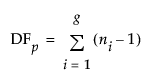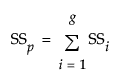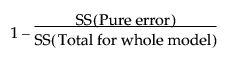Publication date: 11/10/2021

## Lack of Fit Report

#### Pure Error DF

For the Pure Error DF, consider the cases where more than one observation has the same value for height. In general, if there are g groups having multiple rows with identical values for each effect, the pooled DF, denoted DFp, is defined as follows:where ni is the number of observations in the ith group.

#### Pure Error SS

For the Pure Error SS, in general, if there are g groups having multiple rows with the same x value, the pooled SS, denoted SSp, is defined as follows:where SSi is the sum of squares for the ith group corrected for its mean.

#### Max RSq

Because Pure Error is invariant to the form of the model and is the minimum possible variance, Max RSq is calculated as follows: